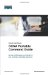# Converting Between Decimal Numbers and Binary

In any given octet of an IP address, the 8 bits can be defined as follows:

 27 26 25 24 23 22 21 20 128 64 32 16 8 4 2 1

To convert a decimal number into binary, you must turn on the bits (make them a 1) that would add up to that number, as follows:

187 = 10111011 = 128+32+16+8+2+1
224 = 11100000 = 128+64+32

To convert a binary number into decimal, you must add the bits that have been turned on (the 1s), as follows:

10101010 = 128+32+8+2 = 170
11110000 = 128+64+32+16 = 240

The IP address 138.101.114.250 is represented in binary as:

10001010.01100101.01110010.11111010

The subnet mask of 255.255.255.192 is represented in binary as:

11111111.11111111.11111111.11000000CCNA Portable Command Guide
ISBN: 1587201585
EAN: 2147483647
Year: 2006
Pages: 261
Authors: Scott Empson

Similar book on Amazon

flylib.com © 2008-2017.
If you may any questions please contact us: flylib@qtcs.net